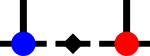SyTen## ◆ EliminateZeros

 strong

Whether to eliminate zeros in tensor products.

Specifically, in each dense sub-block, it compares the element n_ij, calculated as

$$n_{ij} = \sum_k a_{ik} b_{kj}$$

against a threshold times the absolute values squared, i.e. if

$$n_{ij} < \epsilon \times \left(\sum_k |a_{ik}| |b_{ik}|\right)$$

then $$n_{ij}$$ is set to zero.